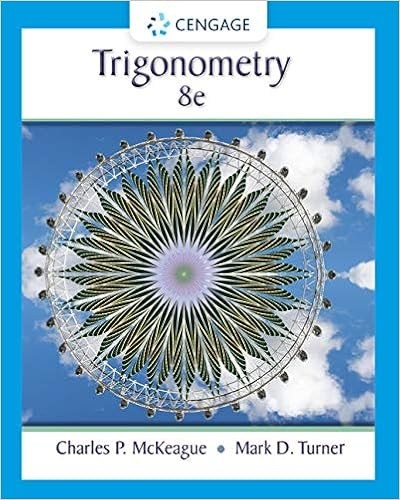# Subtract to get the relief 6 the relief of this map

• Lab Report
• 14
• 55% (11) 6 out of 11 people found this document helpful

This preview shows page 6 - 10 out of 14 pages.

##### We have textbook solutions for you!
The document you are viewing contains questions related to this textbook.The document you are viewing contains questions related to this textbook.
Chapter 7 / Exercise 52
Trigonometry
McKeague/TurnerExpert Verified
Subtract to get the relief =________ 6. The relief of this map is: a. 200 feet b. 280 feet c. 360 feet d. 400 feet
7. If the river on this map is 1.93 miles long, what is the gradient for this river?
Hint: Gradient is Rise / Run Rise = Contour difference of the river = ?_____ Feet Run = Length of the river (given) = ______ Miles Therefore, gradient = ?
##### We have textbook solutions for you!
The document you are viewing contains questions related to this textbook.The document you are viewing contains questions related to this textbook.
Chapter 7 / Exercise 52
Trigonometry
McKeague/TurnerExpert Verified
8. The steepest slope is found between:
9. The elevation of point “A” is:
10. The elevation of point “B” is: a. 40 feet b. 60 feet c. 80 feet d. 120 feet e. 160 feet f. 200 feet
11. The elevation of point “C” is:
12. The elevation of point “F” is:
13. A person standing at point “F” would only be visible to a person standing at:
A-A’ cuts 15 contours line.-FIRST FILL IN THE 15 YELLOW BOXES WITH CONTOUR VALUES STARTING using correct contour interval. -Then each of these points need to be placed in their correct elevation on the PROFILE BELOW.
•••